# Visual Basic Loops, IF, ELSE and ELSEIF statements and creating ranges in Visual Basic 6 for Excel 2007 and Excel 2010

## Using FOR and NEXT to create loops, Ranges to group cells and IF, ELSE and ELSEIF statements in Visual Basic for Excel 2007 and Excel 2010

Hi and welcome to my second hub on Visual Basic or Visual Basic for Applications. Today, we will learn how to do three very important things that you will find essential when writing code in VB:

• Defining cell ranges
• Creating loops using FOR and NEXT
• Working with IF statements using AND and THEN as well as working with ELSE and ELSEIF

Ranges allow you to define a group of cells which when used with a loop will enable you to do something to each cell in the range. In today’s example, I fill each cell in the range with a colour based on its value.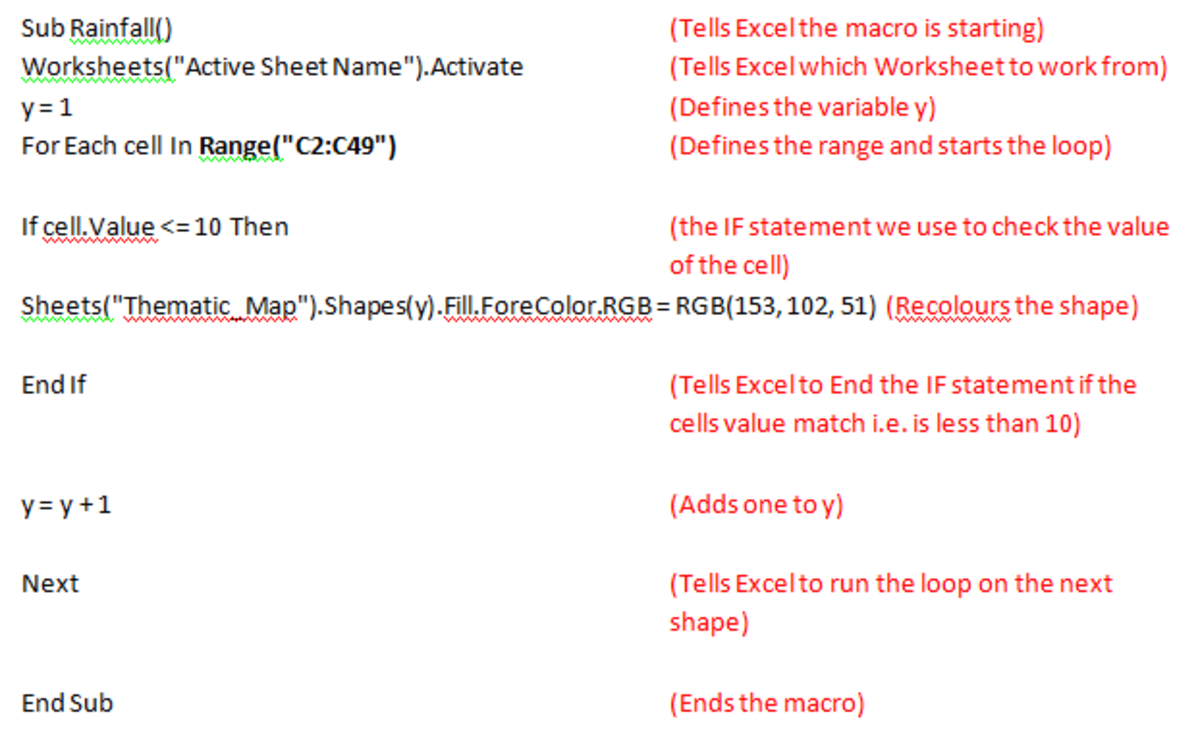Example of loops, ranges and FOR NEXT statements used in Visual Basic code using Excel 2007 and Excel 2010.http://robbiecwilson.hubpages.com/

FOR and NEXT statements are used to create loops which you can then repeat a specific number of times. In today’s example, I use a loop to fill the foreground of each shape, starting with Shape Number 1, then, 2, 3… until the last Shape after which the code finishes.

IF works in the same way that the IF function works when you use it in formulas in Excel. For Example:

IF cell.Value <= 29.9 AND cell.Value >= 20 THEN

To learn more about IF as well as how to use the logical operators, AND, OR and NOT alongside IF, I have a hub that covers this very versatile and powerful function in greater detail.

http://robbiecwilson.hubpages.com/hub/Using-the-IF-and-IFERROR-functions-as-well-as-the-logical-functions-AND-OR-and-NOT-in-Excel-2007-and-Excel-2010

I introduce the basics of Visual Basic or VBA code in the following hub; including how to create new code, how to start and finish it, how to troubleshoot errors and the basic code you will need to know to work with VB:

http://robbiecwilson.hubpages.com/hub/Visual-Basic-for-Excel-2007-and-Excel-2010-A-Beginners-Guide

The code that I wrote for both of my introductory hubs on Visual Basic was used to create a thematic map in Excel, which allows you to display variations across geographical regions on a map (for example population density across America). My hub on how to create a thematic map can be found here:

http://robbiecwilson.hubpages.com/hub/How-to-create-a-thematic-or-Choropleth-map-in-Excel-2007-and-Excel-2010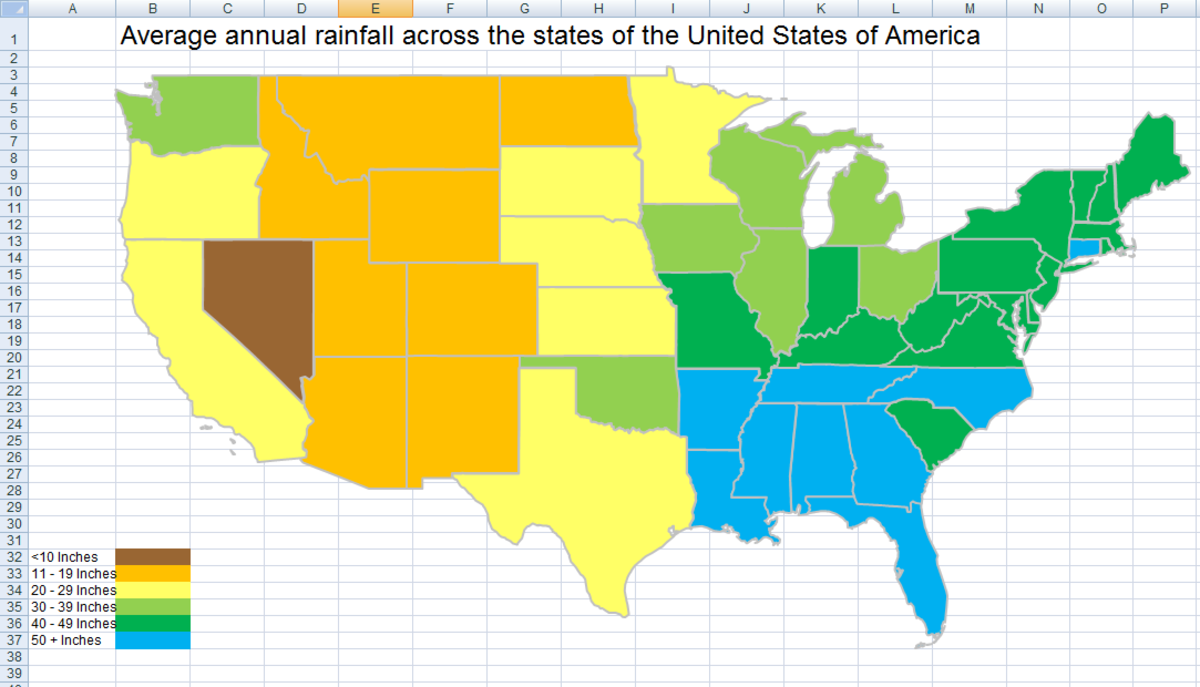Thematic map created using Visual Basic code in Excel 2007 and Excel 2010.http://robbiecwilson.hubpages.com/

In addition, I used UserForms and Visual Basic code alongside Command Buttons, Combo Boxes, Option or Radio Buttons and Slide Bars to create a Hotel Reservation User Interface that automatically enters the users input into an Excel worksheet. That hub can be found here:

http://robbiecwilson.hubpages.com/hub/User-Interface-design-using-a-UserForm-in-Excel-2007-and-Excel-2010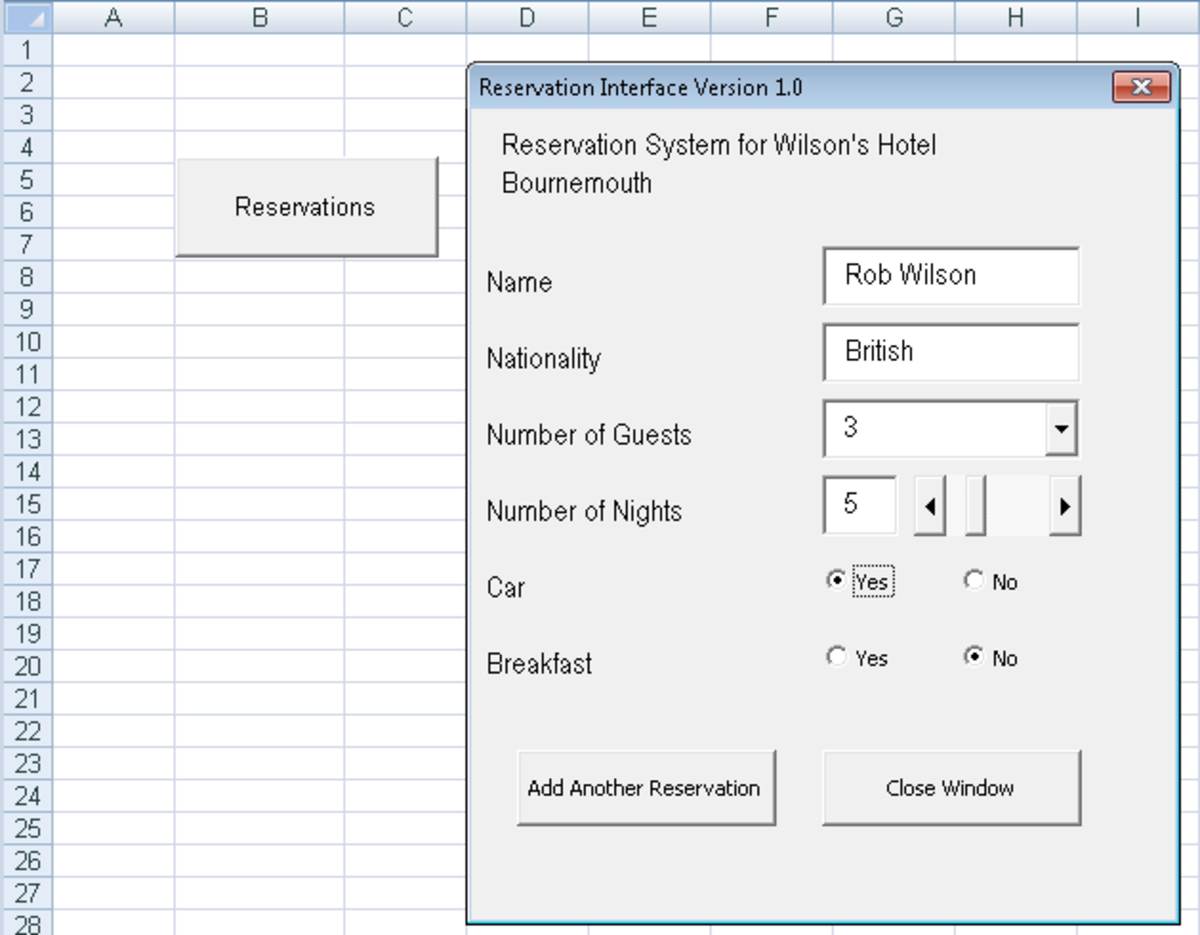Hotel Reservation User Interface created using UserForms and Visual Basic code in Excel 2007 and Excel 2010.http://robbiecwilson.hubpages.com/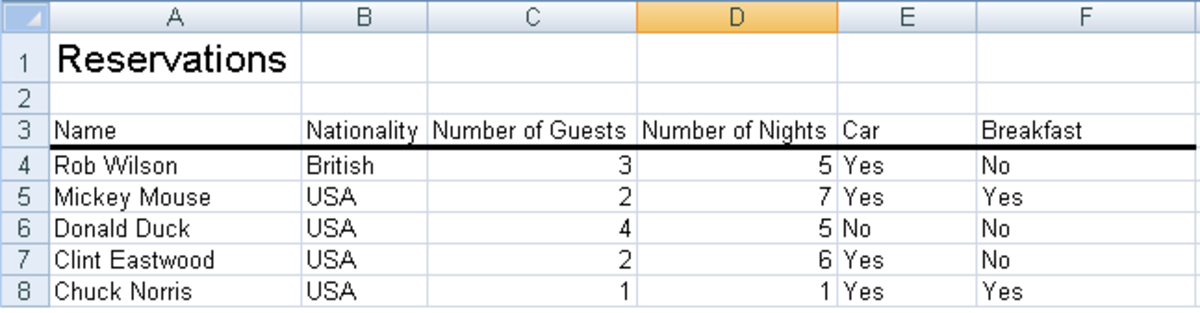Example of reservations using the above User Interface, created automatically in a worksheet in Excel 2007 or Excel 2010.http://robbiecwilson.hubpages.com/

## Creating a range in Visual Basic in Excel 2007 and Excel 2010

Ranges allow you to group an area of cells together so that you can perform a task on each cell in the range. There are two types of range:

• A defined range where you know exactly what cells you want to include for which you will use Range
• An undefined range is when you do not know exactly what cells you want to include, or the range is dynamic in which case you will use the command CurrentRegion
Scroll to Continue

The syntax for a defined range is bolded in the figure below:

Range("C2:C49")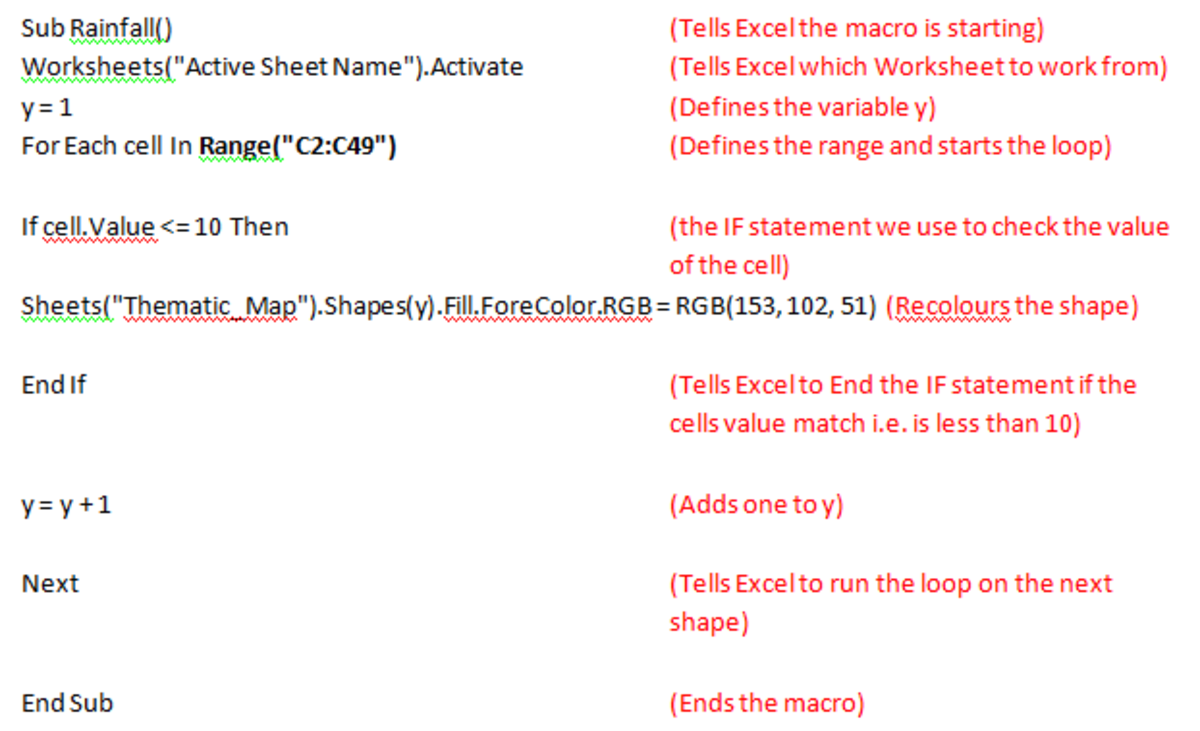Visual Basic code used to define a range of cells in Excel 2007 and Excel 2010.http://robbiecwilson.hubpages.com/

The syntax for an undefined range uses CurrentRegion and ActiveCell to tell Excel to perform something to all the cells in the area near the active cell.

To activate a specific cell: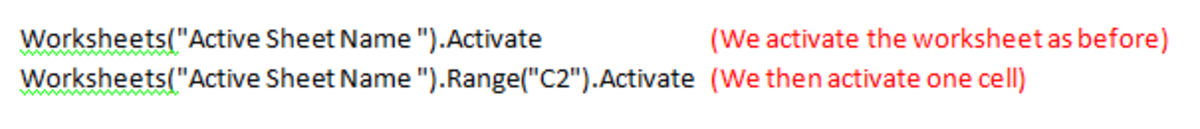Visual Basic code used to activate specific cells in Excel 2007 and Excel 2010.http://robbiecwilson.hubpages.com/

Then we can use the active cell to tell Excel to do something to every cell in the region near our active cell C2.

For Each cell In ActiveCell.CurrentRegion

This will start a loop where Excel will do the task that we define to each cell in the area near our active cell.

## Creating Loops using Visual Basic in Excel 2007 and Excel 2010

To create a loop in VB, we use the FOR and NEXT functions. In addition, you use a variable to tell Excel how many times to run the loop. I have typed in bold the code that makes up our loop below: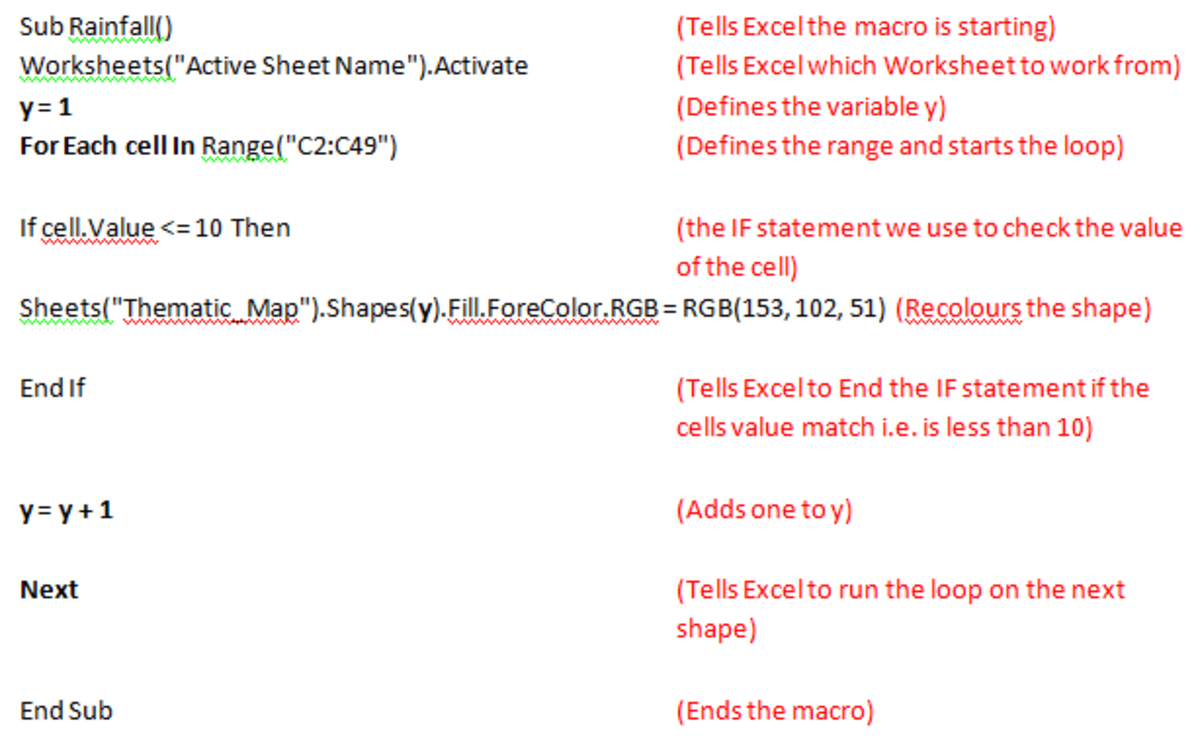Example of a Visual Basic loop in Excel 2007 and Excel 2010.http://robbiecwilson.hubpages.com/

The first line:

Y=1

Sets our variable that we will use to determine the number of times the loop will run.

For Each cell In Range(“C2:C49”)

Looks at each cell in that range in order and checks it against the IF statement.

Sheets("Thematic_Map").Shapes(y).

Starts with Shape Number 1 as Y = 1 the first time the loop is run.

Y=y+1

Adds one to Y, Y becomes 2, then 3 and 4 until there are no more shapes.

Next

Tells Excel to rerun the loop as long as there are shapes to colour and cells to check

Note: Each FOR statement needs a NEXT statement to complete the routine. Your code will not work without both being present and Excel will give you the following error.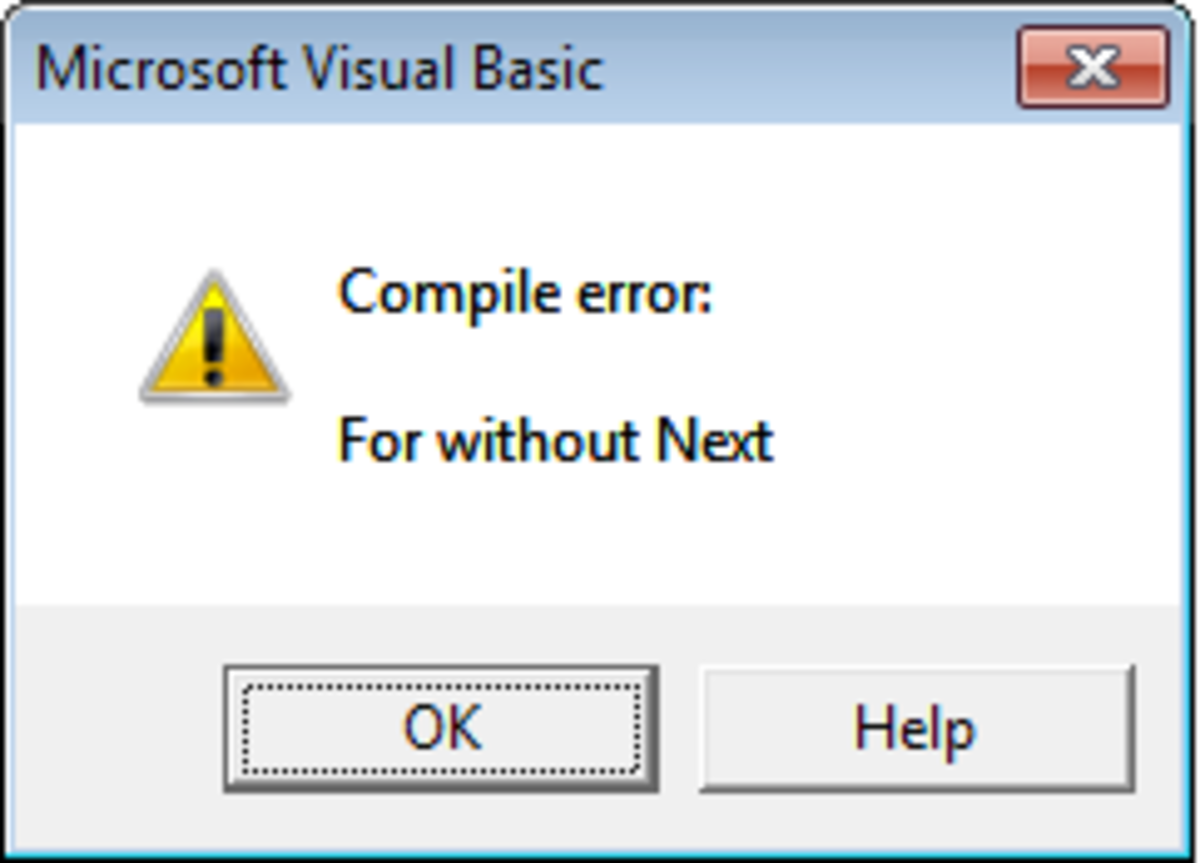Example of the error received if a complete FOR NEXT statement is not present in Excel 2007 or Excel 2010 Visual Basic.http://robbiecwilson.hubpages.com/

## Using IF statements in Visual Basic for Excel 2007 and Excel 2010

The final part of our code is to use an IF statement. As with using IF in formulas, you can use IF with logical operators such as AND and THEN.

Note: For every IF statement, you need an END IF to finish the code.

If cell.Value <= 29.9 And cell.Value >= 20 Then

Sheets("Thematic_Map").Shapes(y).Fill.ForeColor.RGB = RGB(255, 255, 102)

End If

As before, the parts that pertain to the IF statement are in bold. What the code is doing is:

If the value of the cell is less than 29.9 and more than 20 then Excel will do what is on the next line.

To illustrate this with an example, if the cell that Excel is looking at is 15 then Excel will not do anything. If the cell is 21, then it will re-colour that cell as it is between 20 and 29.9

As you can see from the figure below, you can have a number of IF statements one after the other. Excel will work through each IF statement until it finds one that matches the cell (or variable) you are asking it to compare them with.

Note: If the cell contents (or variable) do not match what is being looked for in any of the IF statements (a number above 70 in my code for example) then Excel will do nothing to that cell.

## Using IF with ELSE and ELSEIF in Visual Basic for Excel 2007 and Excel 2010

Rather than using a number of IF statements as we did above, you can use ELSE and ELSEIF commands instead. Working with the same IF statements as we did above, you can see the code using ELSEIF and also ELSE.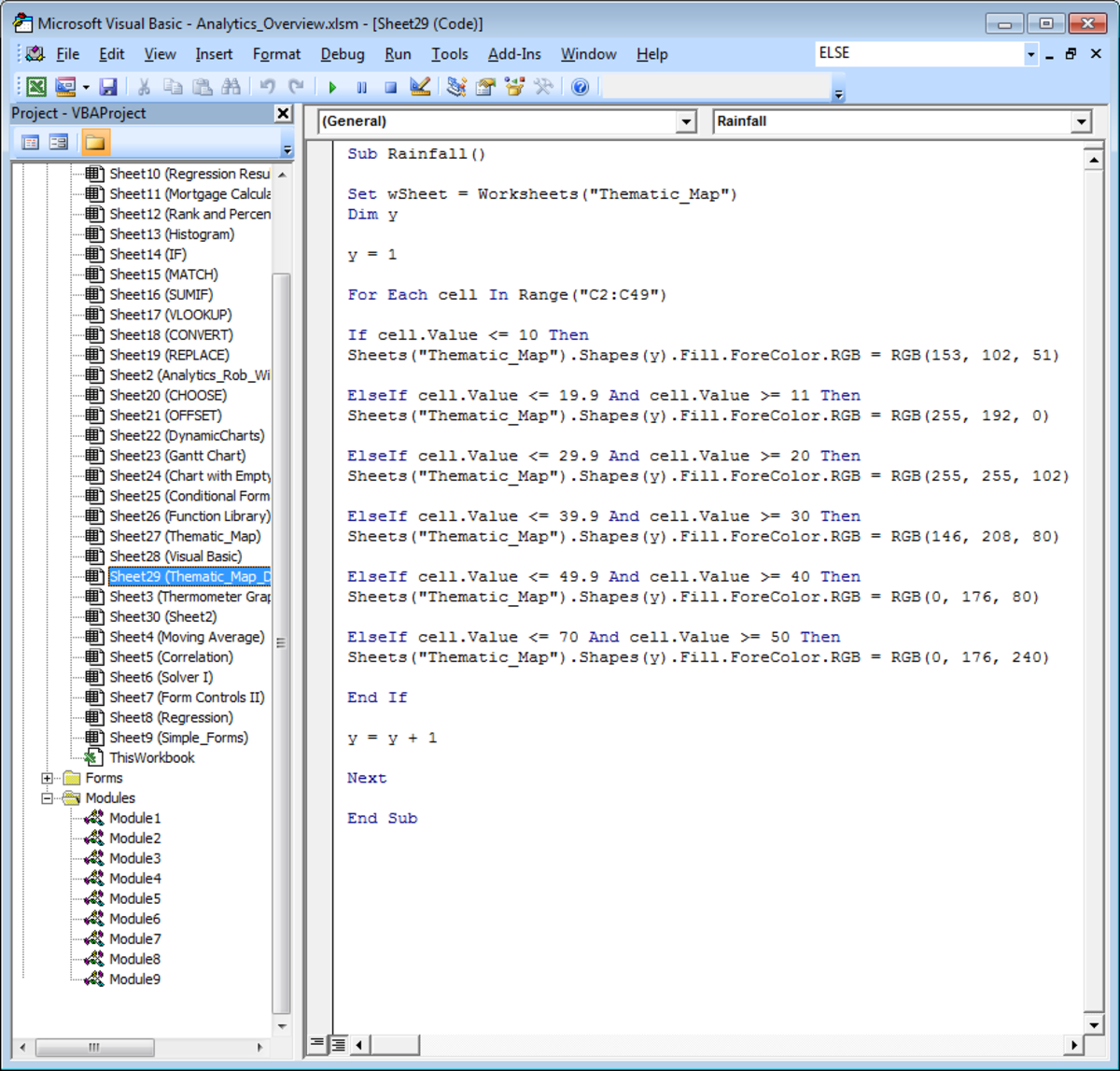Example of using the ELSEIF Visual Basic command in Excel 2007 and Excel 2010.http://robbiecwilson.hubpages.com/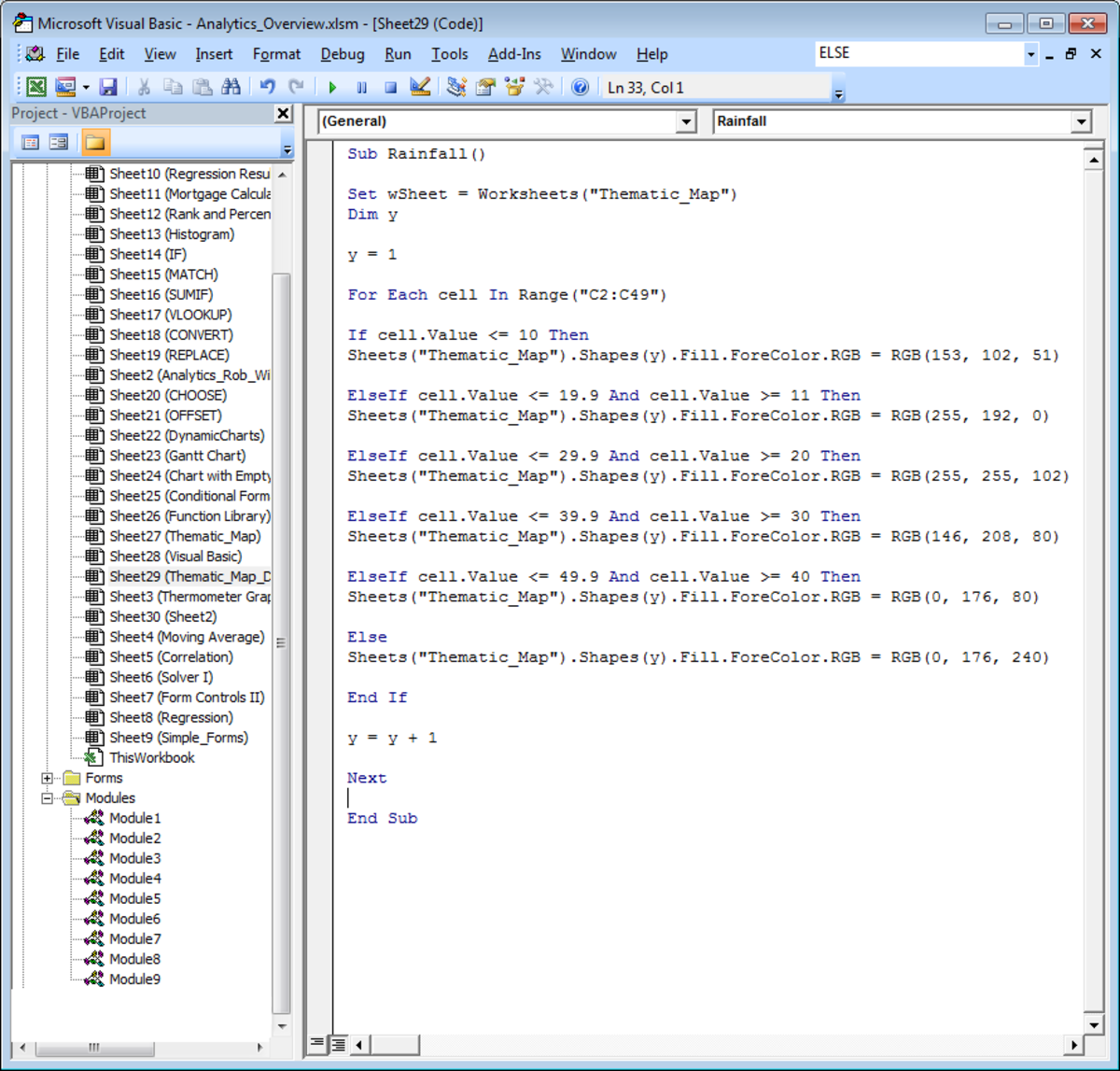Using the ELSE command in Visual Basic in Excel 2007 and Excel 2010.http://robbiecwilson.hubpages.com/

Note: ELSE is excellent as a catch all, in the example shown above, it will fill all cells above 50, whereas the ELSEIF will only fill cells between 50 and 70, any shapes linked to cells with higher values will remain blank.

Note: You cannot use ELSE directly with an IF command (shown in the figure below). ELSE should be used at the end of a bank of IF or ELSEIF statements to work as a catch all to ensure that all values outside the previous IF or ELSEIF statements are dealt with in some way.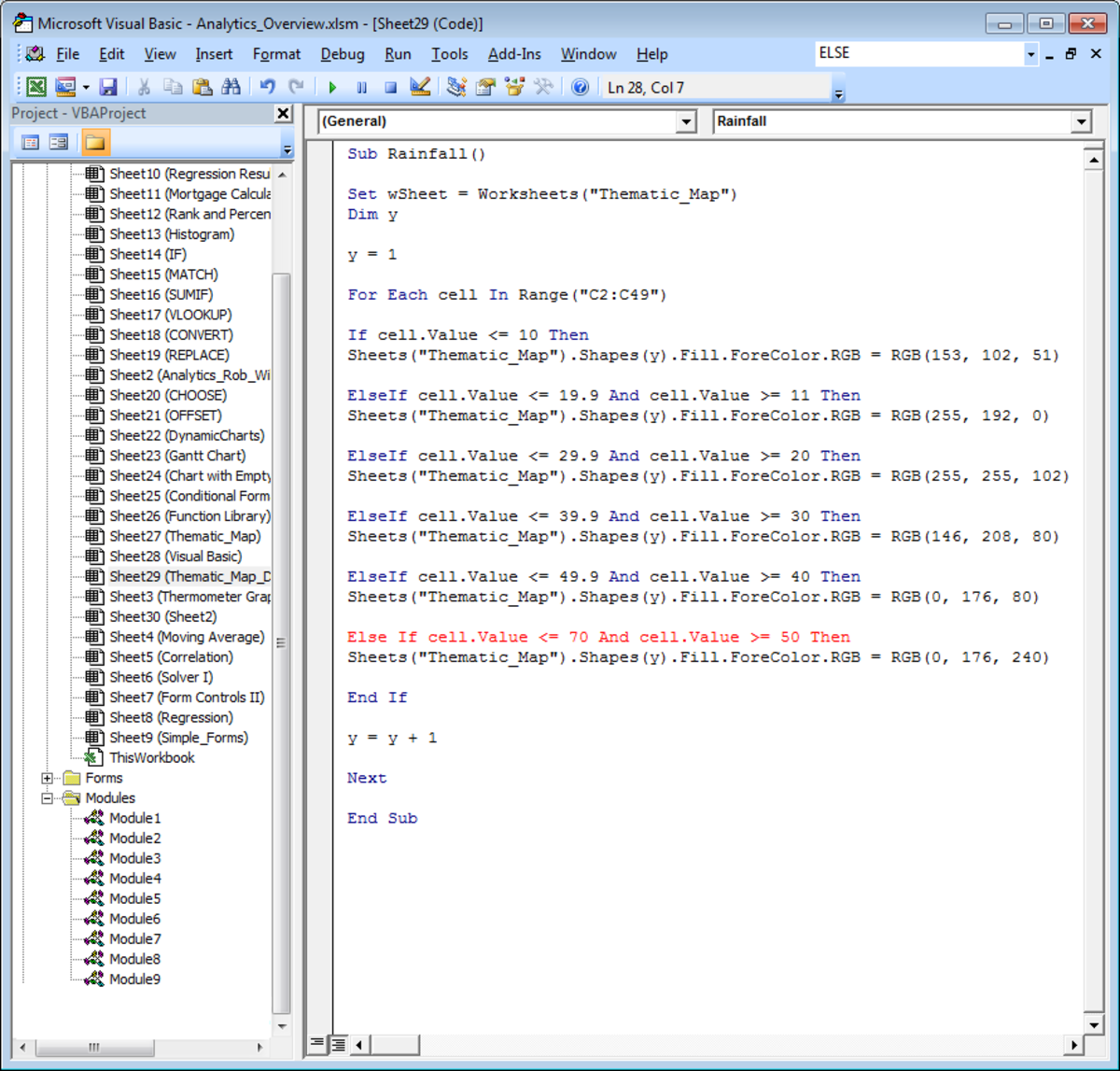ELSE and IF cannot be used in the same line of Visual Basic code in Excel 2007 and Excel 2010.http://robbiecwilson.hubpages.com/

## Conclusion

Ranges, loops using FOR NEXT statements and IF / ELSEIF statements are very important for anyone who wants to create code using Visual Basic to understand and to be able to use. In this hub, we worked through:

• Creating a range for defined and undefined or dynamic data
• Constructing FOR, NEXT loops
• Using IF statements
• Using ELSE and ELSEIF with IF

Armed with these important concepts, the code that you can now create will be far more useful and powerful than before. You will be able to, as I was when I created this code, easily manipulate a large amount of data in a flexible and controlled manner. Good luck with creating code in VB to make your life easier and your data more useful and powerful. Many thanks for reading, please feel free to leave any comments you many have below.